# Chapter 10: Ordinary Differential Equations - Online Test

Q1. The degree of the differential equation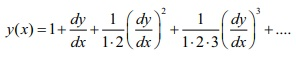is
Explaination / Solution: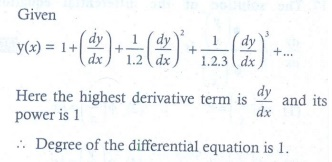Q2. If p and q are the order and degree of the differential equation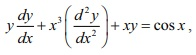when
Explaination / Solution: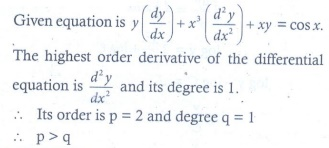Q3. The solution of the differential equation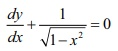is
Explaination / Solution: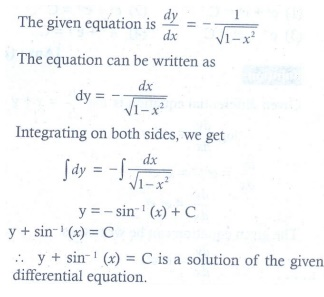Q4. The solution of the differential equation dy/dx = 2xy is
Explaination / Solution: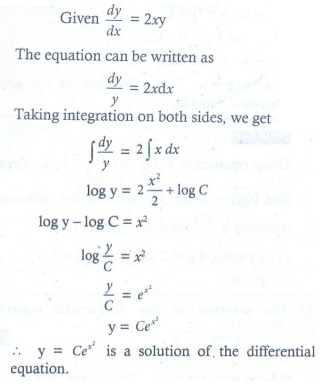Q5. The general solution of the differential equation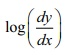= x + y is
Explaination / Solution: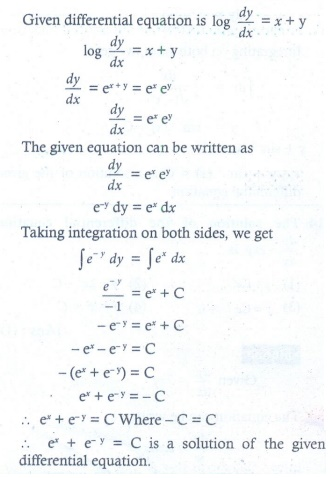Q6. The solution of dy/dx = 2y −x is
Explaination / Solution: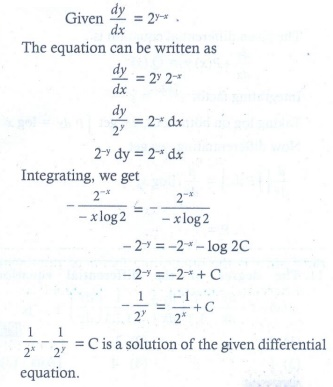Q7. The solution of the differential equation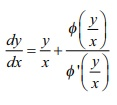is
Explaination / Solution: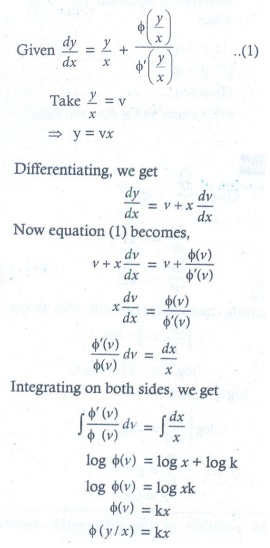Q8. If sin x is the integrating factor of the linear differential equation dy/dx+ Py = Q , then P is
Explaination / Solution: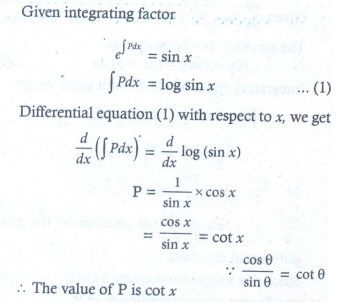Q9. The number of arbitrary constants in the general solutions of order n and n +1are respectively
Explaination / Solution: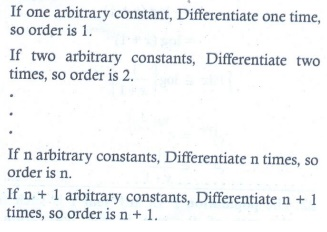Q10. The number of arbitrary constants in the particular solution of a differential equation of third order is
Explaination / Solution:
No Explaination.